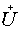# On isometric dilations of commutative systems of linear operators

## Ключові слова:

dilation, commutative systems of linear operators.

## Анотація

The isometric dilation of two parameter semigroup T(n) = T1n1 T2n2, where n = (n1, n2) Î Z2+, for a commutative system {T1, T2} of linear bounded operators, one of which is a contraction, ||T1|| ≤ 1, is constructed. The building of the dilation is based on characteristic qualities of the com- mutative isometric expansionwhich was given in the previous work by the author . The isometric dilations U(n) and(n) of the semigroups T(n) and T*(n) are shown to be unitarily linked.

Mathematics Subject Classification: 47A45.

## Як цитувати

(1)
Zolotarev, V. On Isometric Dilations of Commutative Systems of Linear Operators. J. Math. Phys. Anal. Geom. 2005, 1, 192-208.

## Розділ

Статті

### Завантаження

Дані завантаження ще не доступні.